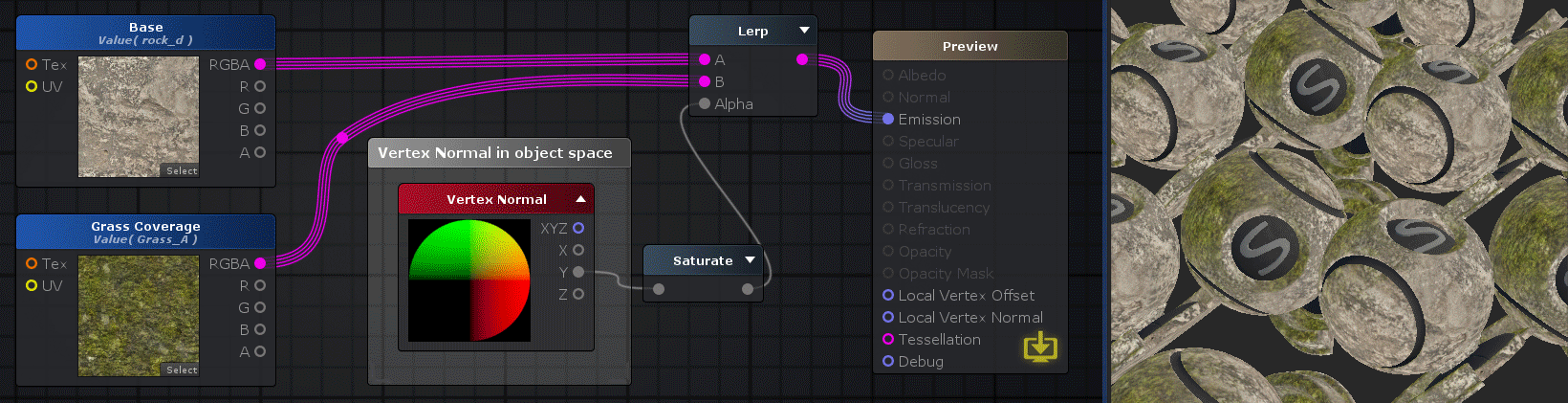# Unity Products:Amplify Shader Editor/Vertex Normal

## Vertex Normal Node

The Vertex Normal Node outputs the vertices normal vectors in object space. This data is extracted directly from the mesh and contains the vertex normal relative to the object origin, this means the normal vector values don't change whatever transform value your game object has. This is usually useful to use in the Local Vertex Normal output or to create effects that are bound to the object and stay exactly the same even if your game object changes position, rotation or size.
Depending on where this node is being used ( vertex or fragment/surface function ) it will either return a direct value ( on vertex functions ) or an interpolated value between vertices( on fragment/surface functions ).

NOTE: Do not confuse with World Normal node.Nodes used: Texture Sample, Vertex Normal, Saturate, Lerp

Output Port Description Type
XYZ Returns the vertex normal vector in object space Float3(4)
X Returns only the X component of the vertex normal Float
Y Returns only the Y component of the vertex normal Float
Z Returns only the Z component of the vertex normal Float